Author: Oscar Cronquist Article last updated on February 23, 2019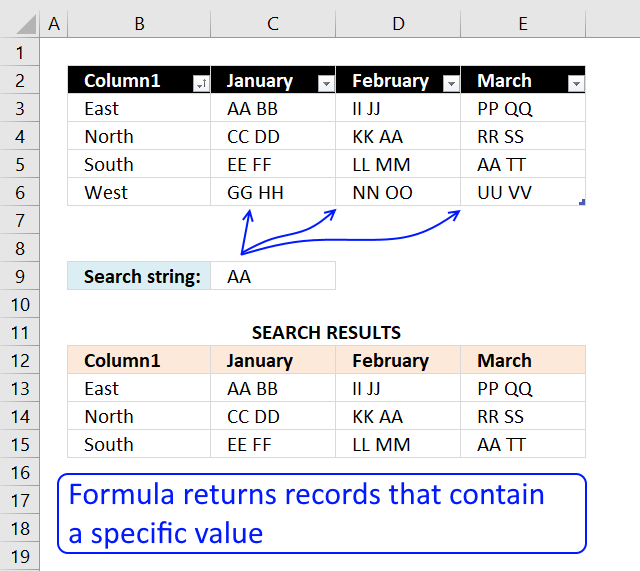This article explains different techniques that filter rows/records that contain a given text string in any of the cell values in a record. Example, row 3 is extracted above because string AA is found in cell C3. Row 4 and 5 are also extracted because string AA is found in cell D4 and E5 respectively.

How do I extract rows that contain a string in a data set or table?
You can use a formula to extract records based on a search value, it also returns multiple records if there are any that match. The advantage of using a formula is that it is dynamic meaning the result changes as soon as a new search value is entered. The downside with the formula is that it may become slow if you have lots of data to work with.

How do I filter rows that contain a string using Advanced Filter?
You also have the option to filter records using an Advanced Filter, it allows you to perform multiple search values using OR-logic across multiple columns. This article explains how to set it up, jeep in mind that it needs a small amount of manual work in order to apply new filters.

How do I filter rows that contain a string using an Excel defined Table?
The Excel defined Table needs the COUNTIF function to accomplish the task which may slow down the calculation considerably if your data set is huge. I recommend using the Advanced Filter if speed is an issue.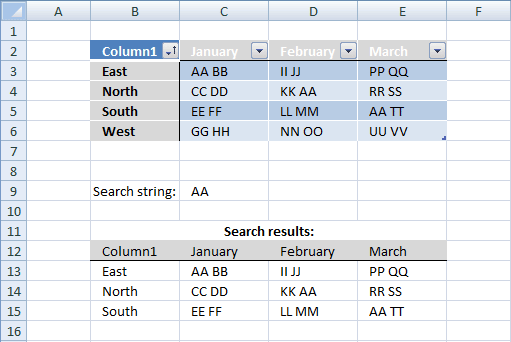Array formula in B13:

=IFERROR(INDEX(Table1, SMALL(IF(ISNUMBER(FIND(\$C\$9, Table1)), MATCH(ROW(Table1), ROW(Table1)), ""), ROWS(\$A\$1:A1)), COLUMNS(\$A\$1:A1)), "")

To enter an array formula, type the formula in cell B13 then press and hold CTRL + SHIFT simultaneously, now press Enter once. Release all keys.

The formula bar now shows the formula with a beginning and ending curly bracket telling you that you entered the formula successfully. Don't enter the curly brackets yourself.

Copy cell B13 and paste to cell range B13:E16. Replace FIND function with SEARCH function if you don't want the formula to perform a case sensitive search.

### Explaining array formula in cell B13

#### Step 1 - Identify cells containing search string

The FIND function returns the starting point of one text string within another text string, it returns a number representing the position of the found string. If not found the function returns the #VALUE! error.

FIND(\$C\$9, \$B\$3:\$E\$6)

returns

{#VALUE!, 1, #VALUE!, #VALUE!; #VALUE!, #VALUE!, 4, #VALUE!; #VALUE!, #VALUE!, #VALUE!, 1; #VALUE!, #VALUE!, #VALUE!, #VALUE!}

The ISNUMBER function returns TRUE if the value in the array is a number and FALSE if not a number, it returns FALSE even if the value is an error value which is handy in this case.

ISNUMBER(FIND(\$C\$9, \$B\$3:\$E\$6)) creates this array displayed in cell B11:E14: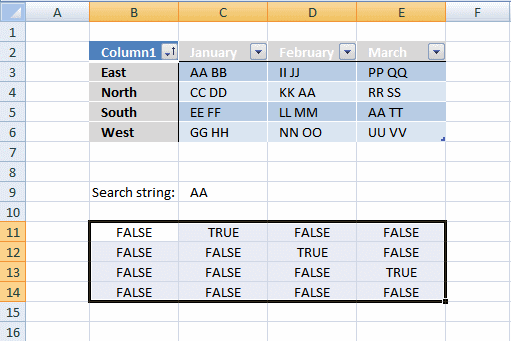Column B has no cells containing string "AA".

Column C has 1 cell containing string "AA". Cell C3

Column D has 1 cell containing string "AA". Cell D4.

Column E has 1 cell containing string "AA". Cell E5.

#### Step 2 - Return row number

We need to calculate the row number for each cell in order to replace TRUE in the array with the corresponding row number. To create the array we need we use the MATCH function and the ROW function.

MATCH(ROW(Table1), ROW(Table1)) returns this array displayed in cell range B11:E14:If string "AA" is found in a cell in the table the corresponding row number is returned.

#### Step 3 - Replace boolean values with row numbers

The IF function has three arguments, the first one must be a logical expression. If the expression evaluates to TRUE then one thing happens (argument 2) and if FALSE another thing happens (argument 3).

IF(ISNUMBER(FIND(\$C\$9, Table1)), MATCH(ROW(Table1), ROW(Table1)), "")

becomes

IF({#VALUE!, 1, #VALUE!, #VALUE!; #VALUE!, #VALUE!, 4, #VALUE!; #VALUE!, #VALUE!, #VALUE!, 1; #VALUE!, #VALUE!, #VALUE!, #VALUE!}, MATCH(ROW(Table1), ROW(Table1)), "")

becomes

IF({#VALUE!, 1, #VALUE!, #VALUE!; #VALUE!, #VALUE!, 4, #VALUE!; #VALUE!, #VALUE!, #VALUE!, 1; #VALUE!, #VALUE!, #VALUE!, #VALUE!}, {1; 2; 3; 4}, "")

and returns the following array shown in cell range B11:E14: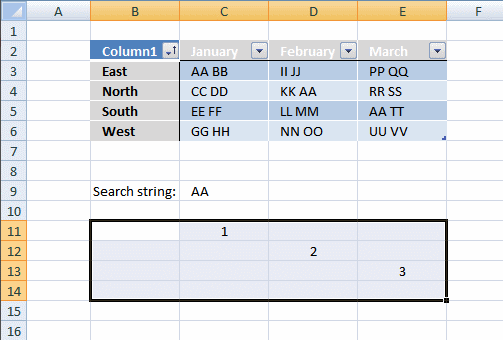#### Step 4 - Sort the row numbers from smallest to largest

To be able to return a new value in a cell each I use the SMALL function to filter column numbers from smallest to largest.

The ROWS function keeps track of the numbers based on an expanding cell reference. It will expand as the formula is copied to the cells below.

SMALL(IF(ISNUMBER(FIND(\$C\$9, Table1)), MATCH(ROW(Table1), ROW(Table1)), ""), ROWS(\$A\$1:A1))

returns 1.

#### Step 5 - Return a value at the intersection of a particular row and column

The INDEX function returns a value based on a cell reference and column/row numbers.

INDEX(Table1, SMALL(IF(ISNUMBER(FIND(\$C\$9, Table1)), MATCH(ROW(Table1), ROW(Table1)), ""), ROWS(\$A\$1:A1)), COLUMNS(\$A\$1:A1))

becomes

INDEX(Table1, 1, COLUMNS(\$A\$1:A1))

becomes

INDEX(Table1, 1, 1)

and returns "East" in cell B13.

#### Step 6 - Remove errors

The IFERROR function allows you to display a blank if the formula returns an error.

Note, the IFERROR function handles all errors. Use with care.

## Search for a text string in a data set and return multiple records [Excel defined Table]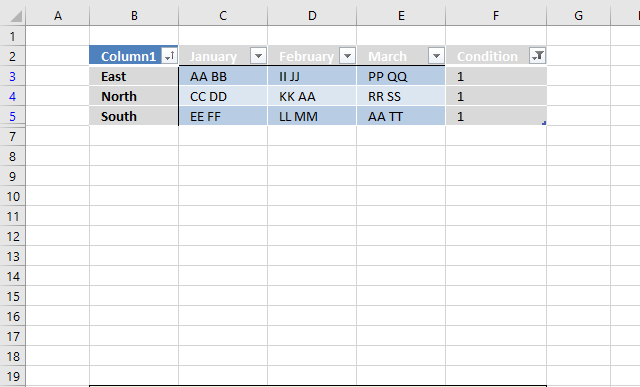### Convert dataset to an Excel defined Table

1. Select any cell within the dataset.
2. Press CTRL + T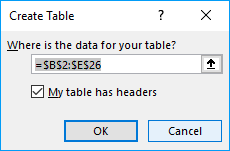4. Click OK button.

### Add formula to Excel defined Table

1. Select cell F3.
2. Type formula: =COUNTIF(Table13[@[January]:[March]],"*AA*")
3. Press Enter.

Excel fills the remaining cells in the table for you and creates a header name for your new column automatically.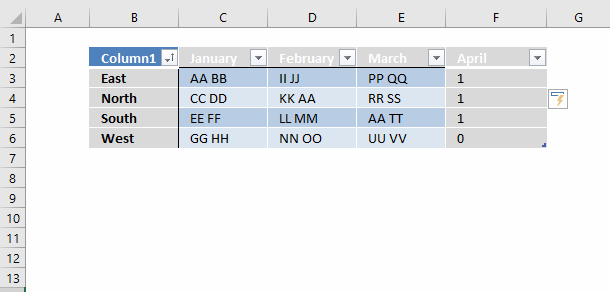### Explaining formula in cell F3

Table13[@[January]:[March]] is a structured reference to data in columns January to March in table Table13.

The COUNTIF function returns 0 (zero) if none of of the cells on the same row contains string AA, to do a wildcard search use the asterisk * before and after the search string. The at sign @ before the header names indicate that the reference is to values on the same row.

COUNTIF(Table13[@[January]:[March]],"*AA*")

becomes

COUNTIF({"AA BB", "II JJ", "PP QQ"}, "*AA*")

and returns 1 in cell F3. String AA was found once in cell range C3:E3.

### Filter Excel defined Table

To filter the records containing string AA at least once follow these steps:

1. Click black arrow next to header name "April".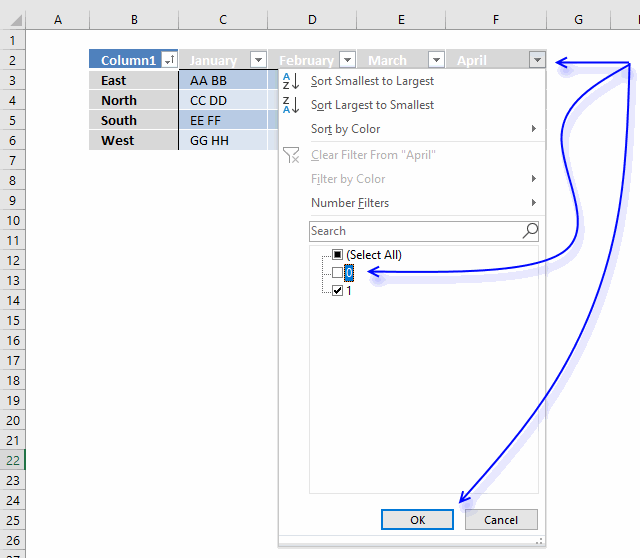2. Click checkbox next to 0 (zero) to deselect it.
3. Click OK button.April is not the correct header name, I changed it to Condition.

## Search for a text string in a data set and return multiple records [Advanced Filter]The Advanced Filter is a powerful feature in Excel that allows you to perform OR-logic between columns. The asterisk lets you do a wildcard lookup meaning that a record is filtered if the text string is found somewhere in the cell value.

1. Copy column headers and paste to cells above or below the dataset.
Note, if you place them next to the dataset they may become hidden when the filter is applied.
2. Type the search condition and add an asterisk before and after the text string.
3. Add another search condition, make sure they are in a row each in order to perform OR-logic.
4. Repeat with remaining criteria.### Apply filter

1. Select the dataset.
2. Go to tab "Data" on the ribbon.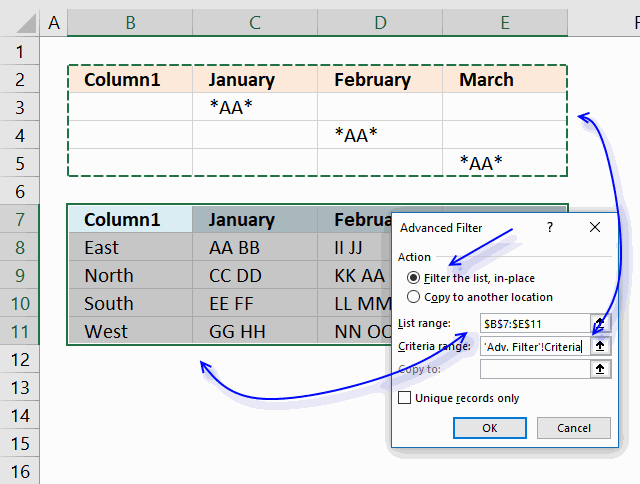4. Click radio button "Filter the list, in-place"
5. Select Criteria range:"
6. Click "OK button.To delete the filter applied simply select a cell within the filtered dataset, then go to tab "Data" on the ribbon and click "Clear" button.

* You will also get a weekly newsletter, unsubscribe anytime!

The following article shows you how to VLOOKUP and return multiple values, the lookup value must match the entire cell value.

5 easy ways to VLOOKUP and return multiple values

This post explains how to lookup a value and return multiple values. No array formula required.

Search for a cell value in a dataset:

Search for a cell value in a dataset

Question: How do I identify rows that have a specific cell value in an Excel defined table? Array formula in […]

How to look for values in a column that contain two text strings:

Search for multiple text strings in column – AND logic

Question: How do I search a list for two text strings and return a list with where both strings occurs? […]

Search two columns for two text strings. The strings can be anywhere in these two columns but both text strings have to be found in different cell values or a single cell value and be on the same row to match:

A record is returned if both search strings are found on the same row

Question: I want to search two columns with two search strings? The strings can be anywhere in these two columns […]

Search for three text strings in three columns, one text string in each column. Return values in which all three cases are found:

Search each column for a string each and return multiple records – AND logic

Question: Can expand this equation set into more than two colums of data, say if I had a first, middle […]

Search for multiple text strings in multiple columns, one text string in each column. Return values in which all text strings match:

Extract records where all criteria match if not empty

Question: I second G's question: can this be done for more than 3? i.e. (Instead of last name, middle, first) […]

Search for a single text string in a single column and return multiple matches.

Search for a text string and return multiple adjacent values

This article demonstrates array formulas that search for cell values containing a search string and returns corresponding values on the […]

Search for a text string in a single column and return multiple corresponding values.

Search for a text string and return multiple adjacent values

This article demonstrates array formulas that search for cell values containing a search string and returns corresponding values on the […]

Search for multiple text strings in a single column and return multiple corresponding values.

Search for a text string and return multiple adjacent values

This article demonstrates array formulas that search for cell values containing a search string and returns corresponding values on the […]

Search for a text string in multiple columns and return corresponding values.

Search for a text string and return multiple adjacent values

This article demonstrates array formulas that search for cell values containing a search string and returns corresponding values on the […]